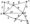# Discrete Mathematical Structures July-2011

1. (a) Show that the following compound propositions are logically equivalent.

(i) p q and ~pvq

(ii) p o q and (p—>q) a [q —> p)

(b) Examine the validity of the following argument.

Px : p^>q,P2: p-±r,Q: p^>(qAr).

2 (a) Show that p —» q logically implies p —> q that is (P q) -> <P q) is a tautology.

(b) Examine the validity of the following argument. “If prices are higher than wages are high. Prices are high or there are price controls. If these are price controls then there is not an inflation. There is an inflation therefore wages are high.

3 (a) Prove that theorem ‘If X is an odd integer, x2 is odd integer.

(b) Prove that in a room of 13 people, 2 or more people have their birthdays in the same month.

4. (a) Show that 1+2+3+—— +n =  for all integer, n≥1 by the principle of mathematical induction.

(B) Prove that 5n+3 is divisible by 4 for all integers n ≥ 0.

5. (a) Define union, intersection and product of two graphs.

(b) A simple graph with n vertices and k components cannot have

(n—k)(n—k +1) more than  edges.

6. (a) Find the minimal spanning tree of the following weighted graph(b) If a connected graph G is Eulerian thenevery vertex of G has even degree.

7. (a) Prove that if A and B are two non empty sets then {AUB)’ = A’∩B’

(b) Define the following functions with examples :

(i) Floor and Ceiling functions

(ii) Div and Mod functions.

8 (a) Prove that if AcB, CcA, BcC then A-B-C

(b) If function f is one-one onto then inverse off i.e. f-1 is also one-one onto.

9 (a) Define group, monoids, semigroups and subgroups.

(b) If G j and G2 are two subgroups of a group G then prove that Gi n G2 is also a subgroup of G.

10  (a) Define cyclic group, permutation group and dihedral group.

(b) Write a short note on coding theory.